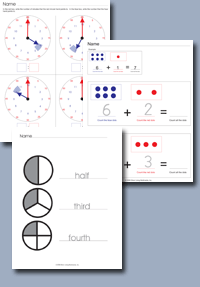﻿ Common Core Standards - Math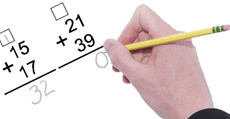Adaptive Worksheets

Helping Every Child to Succeed

toll-free (888) 777-0876
fax (888) 777-0875

## Measurement and Data

#### Measure and estimate lengths in standard units

• 2.MD.A.1.   Measure the length of an object by selecting and using appropriate tools such as rulers, yardsticks, meter sticks, and measuring tapes.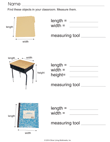Measure Items In The Classroom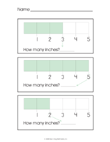Basic Ruler Measurements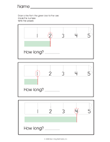Basic Ruler Measurements
• 2.MD.A.2.  Measure the length of an object twice, using length units of different lengths for the two measurements; describe how the two measurements relate to the size of the unit chosen.
• 2.MD.A.3  Estimate lengths using units of inches, feet, centimeters, and meters
• 2.MD.A.4  Measure to determine how much longer one object is than another, expressing the length difference in terms of a standard length unit.

Relate Addition and Subtraction to Length
• 2.MD.B.5  Use addition and subtraction within 100 to solve word problems involving lengths that are given in the same units, e.g., by using drawings (such as drawings of rulers) and equations with a symbol for the unknown number to represent the problem.
• 2.MD.B.6  Represent whole numbers as lengths from 0 on a number line diagram with equally spaced points corresponding to the numbers 0, 1, 2, ..., and represent whole-number sums and differences within 100 on a number line diagram.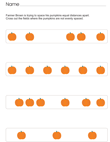Equally Spaced PumpkinsNumber Line Spacing

## Work With Time and Money

• 2.MD.C.7.   Tell and write time from analog and digital clocks to the nearest five minutes, using a.m. and p.m.

Use the following worksheet generator to create custom worksheets.  You can specify the minute intervals.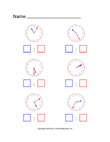Generator
• 2.MD.C.8  Solve word problems involving dollar bills, quarters, dimes, nickels, and pennies, using \$ and ¢ symbols appropriately. Example: If you have 2 dimes and 3 pennies, how many cents do you have?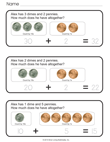Basic Coin Word Problems with Visuals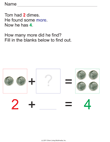Coin Word Problems with Prompts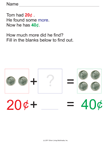Coin Word Problems with Prompts

## Represent and interpret data.

• 2.MD.D.9 Generate measurement data by measuring lengths of several objects to the nearest whole unit, or by making repeated measurements of the same object. Show the measurements by making a line plot, where the horizontal scale is marked off in whole-number units.
• 2.MD.D.10 Draw a picture graph and a bar graph (with single-unit scale) to represent a data set with up to four categories. Solve simple put-together, take-apart, and compare problems1 using information presented in a bar graph.